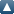# Items where Author is "Jain, MK"Up a level
 Export as ASCII CitationBibTeXDublin CoreEP3 XMLEndNoteHTML CitationJSONMETSMultiline CSVObject IDsOpenURL ContextObjectRDF+N-TriplesRDF+N3RDF+XMLRefWorksReferReference Manager
Group by: Item Type | No Grouping
Number of items: 4.

## Journal article

MOHANTY, R.K., ARORA, U. and JAIN, M.K., 2001. Fourth-order approximation for the three space dimensional certain mildly quasi-linear hyperbolic equation. Numerical Methods for Partial Differential Equations, 17 (3), pp. 277-289. ISSN 0749-159X

MOHANTY, R.K., ARORA, U. and JAIN, M.K., 2001. Linear stability analysis and fourth-order approximations at first time level for the two space dimensional mildly quasi-linear hyperbolic equations. Numerical Methods for Partial Differential Equations, 17 (6), pp. 607-618. ISSN 0749-159X

MOHANTY, R.K., JAIN, M.K. and KUMAR, D., 2001. Single cell discretization of O(kh(2)+h(4)) for the estimates of partial derivative u/partial derivative n for two-space dimensional quasi-linear parabolic equation. Numerical Methods for Partial Differential Equations, 17 (3), pp. 250-261. ISSN 0749-159X

MOHANTY, R.K. and JAIN, M.K., 2001. An unconditionally stable alternating direction implicit scheme for the two space dimensional linear hyperbolic equation. Numerical Methods for Partial Differential Equations, 17 (6), pp. 684-688. ISSN 0749-159X

This list was generated on Sun Oct 24 01:57:53 2021 UTC.